# QuTiP lecture: Superconducting Josephson charge qubits¶

J.R. Johansson ([email protected])

In :
%matplotlib inline
import matplotlib.pyplot as plt
import numpy as np

In :
from qutip import *


### Introduction¶

The Hamiltonian for a Josephson charge qubit is

$\displaystyle H = \sum_n 4 E_C (n_g - n)^2 \left|n\right\rangle\left\langle n\right| - \frac{1}{2}E_J\sum_n\left(\left|n+1\right\rangle\left\langle n\right| + \left|n\right\rangle\left\langle n+1\right| \right)$

where $E_C$ is the charge energy, $E_J$ is the Josephson energy, and $\left| n\right\rangle$ is the charge state with $n$ Cooper-pairs on the island that makes up the charge qubit.

### Helper functions¶

Below we will repeatedly need to obtain the charge qubit Hamiltonian for different parameters, and to plot the eigenenergies, so here we define two functions to do these tasks.

In :
def hamiltonian(Ec, Ej, N, ng):
"""
Return the charge qubit hamiltonian as a Qobj instance.
"""
m = np.diag(4 * Ec * (arange(-N,N+1)-ng)**2) + 0.5 * Ej * (np.diag(-np.ones(2*N), 1) +
np.diag(-np.ones(2*N), -1))
return Qobj(m)

In :
def plot_energies(ng_vec, energies, ymax=(20, 3)):
"""
Plot energy levels as a function of bias parameter ng_vec.
"""
fig, axes = plt.subplots(1,2, figsize=(16,6))

for n in range(len(energies[0,:])):
axes.plot(ng_vec, energies[:,n])
axes.set_ylim(-2, ymax)
axes.set_xlabel(r'$n_g$', fontsize=18)
axes.set_ylabel(r'$E_n$', fontsize=18)

for n in range(len(energies[0,:])):
axes.plot(ng_vec, (energies[:,n]-energies[:,0])/(energies[:,1]-energies[:,0]))
axes.set_ylim(-0.1, ymax)
axes.set_xlabel(r'$n_g$', fontsize=18)
axes.set_ylabel(r'$(E_n-E_0)/(E_1-E_0)$', fontsize=18)
return fig, axes

In :
def visualize_dynamics(result, ylabel):
"""
Plot the evolution of the expectation values stored in result.
"""
fig, ax = plt.subplots(figsize=(12,5))

ax.plot(result.times, result.expect)

ax.set_ylabel(ylabel, fontsize=16)
ax.set_xlabel(r'$t$', fontsize=16);


### Charge qubit regime¶

In :
N = 10
Ec = 1.0
Ej = 1.0

In :
ng_vec = np.linspace(-4, 4, 200)

energies = array([hamiltonian(Ec, Ej, N, ng).eigenenergies() for ng in ng_vec])

In :
plot_energies(ng_vec, energies);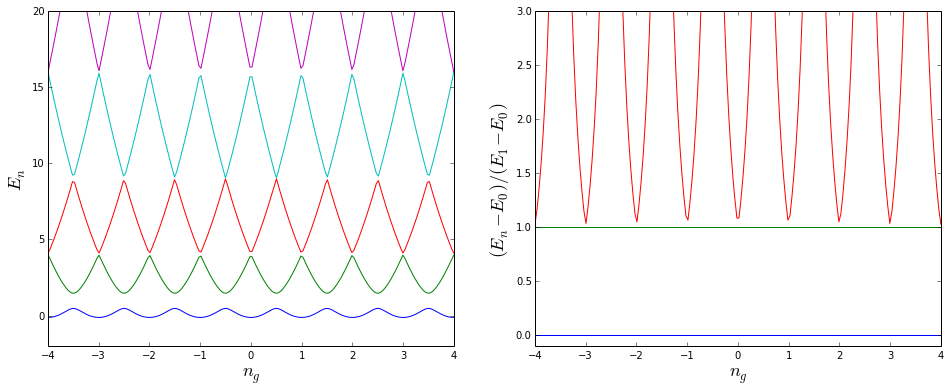In :
ng_vec = np.linspace(-1, 1, 200)

energies = array([hamiltonian(Ec, Ej, N, ng).eigenenergies() for ng in ng_vec])

In :
plot_energies(ng_vec, energies, ymax=(7.5, 3.0));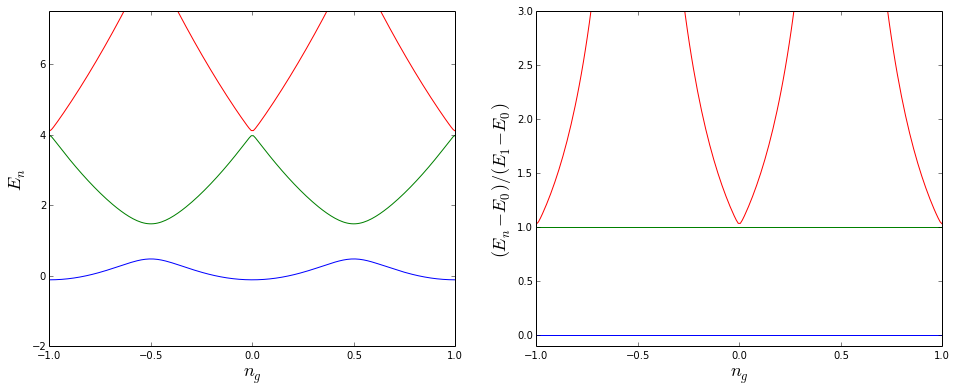### Intermediate regime¶

In :
ng_vec = np.linspace(-4, 4, 200)

In :
Ec = 1.0
Ej = 5.0

In :
energies = array([hamiltonian(Ec, Ej, N, ng).eigenenergies() for ng in ng_vec])

In :
plot_energies(ng_vec, energies, ymax=(50, 3));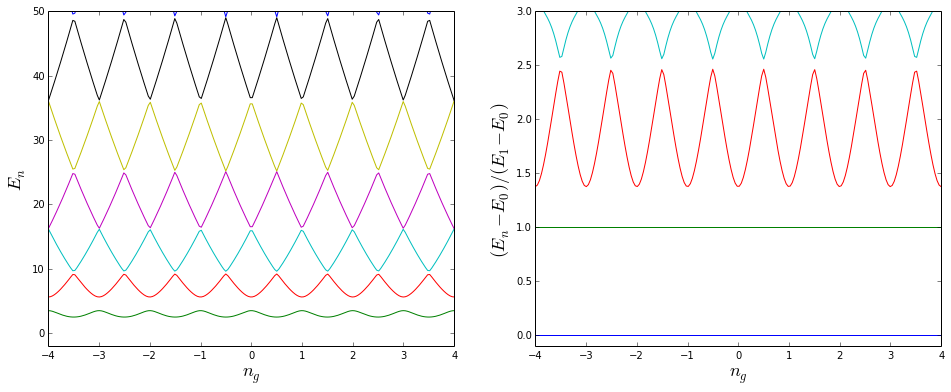In :
Ec = 1.0
Ej = 10.0

In :
energies = array([hamiltonian(Ec, Ej, N, ng).eigenenergies() for ng in ng_vec])

In :
plot_energies(ng_vec, energies, ymax=(50, 3));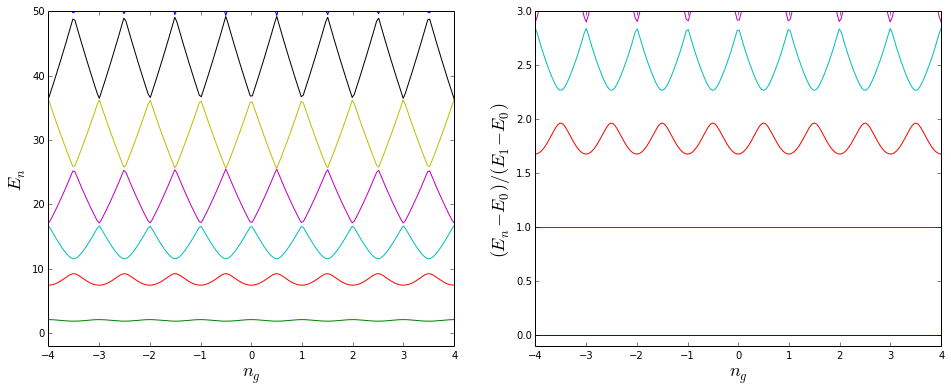### Transmon regime¶

In :
Ec = 1.0
Ej = 50.0

In :
energies = array([hamiltonian(Ec, Ej, N, ng).eigenenergies() for ng in ng_vec])

In :
plot_energies(ng_vec, energies, ymax=(50, 3));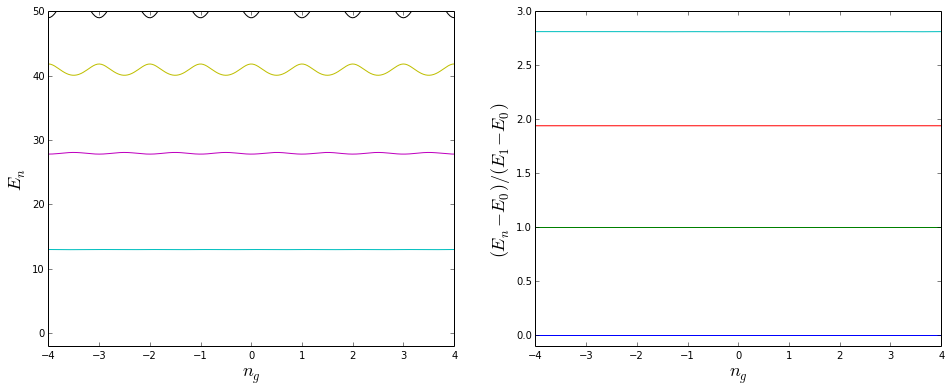Note that the energy-level splitting is essentially independent of the gate bias $n_g$, at least for the lowest few states. This device insensitive to charge noise. But at the same time the two lowest energy states are no longer well separated from higher states (it has become more like an harmonic oscillator). But some anharmonicity still remains, and it can still be used as a qubit if the leakage of occupation probability of the higher states can be kept under control.

## Focus on the two lowest energy states¶

Let's go back to the charge regime, and look at the lowest few energy levels again:

In :
N = 10
Ec = 1.0
Ej = 1.0

In :
ng_vec = np.linspace(-1, 1, 200)

In :
energies = array([hamiltonian(Ec, Ej, N, ng).eigenenergies() for ng in ng_vec])

In :
plot_energies(ng_vec, energies, ymax=(10, 3));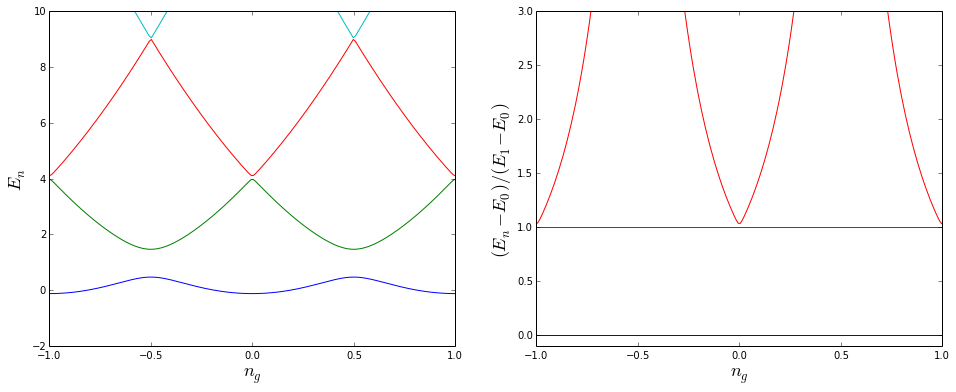We can see that around $n_g = 0.5$ we have two lowest energy levels that are well separated for the higher energy levels:

In :
ng_vec = np.linspace(0.25, 0.75, 200)
energies = array([hamiltonian(Ec, Ej, N, ng).eigenenergies() for ng in ng_vec])
plot_energies(ng_vec, energies, ymax=(10, 1.1));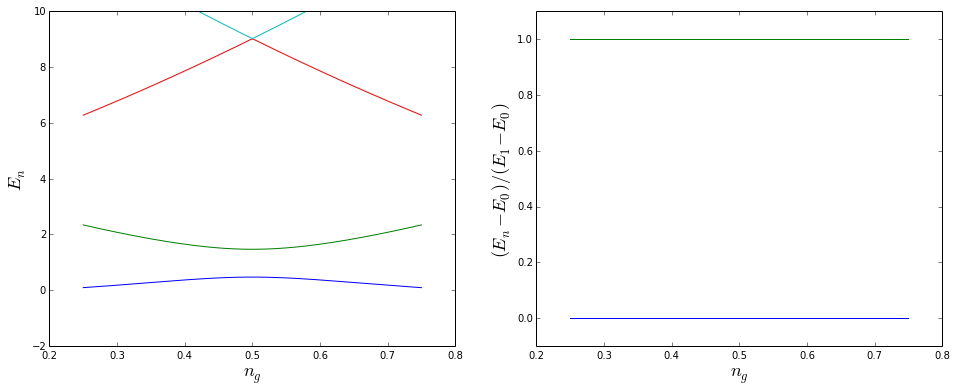Let's tune the system to $n_g = 0.5$ and look at the Hamiltonian and its eigenstates in detail

In :
H = hamiltonian(Ec, Ej, N, 0.5)

In :
H

Out:
Quantum object: dims = [, ], shape = [21, 21], type = oper, isherm = True\begin{equation*}\left(\begin{array}{*{11}c}441.0 & -0.500 & 0.0 & 0.0 & 0.0 & \cdots & 0.0 & 0.0 & 0.0 & 0.0 & 0.0\\-0.500 & 361.0 & -0.500 & 0.0 & 0.0 & \cdots & 0.0 & 0.0 & 0.0 & 0.0 & 0.0\\0.0 & -0.500 & 289.0 & -0.500 & 0.0 & \cdots & 0.0 & 0.0 & 0.0 & 0.0 & 0.0\\0.0 & 0.0 & -0.500 & 225.0 & -0.500 & \cdots & 0.0 & 0.0 & 0.0 & 0.0 & 0.0\\0.0 & 0.0 & 0.0 & -0.500 & 169.0 & \cdots & 0.0 & 0.0 & 0.0 & 0.0 & 0.0\\\vdots & \vdots & \vdots & \vdots & \vdots & \ddots & \vdots & \vdots & \vdots & \vdots & \vdots\\0.0 & 0.0 & 0.0 & 0.0 & 0.0 & \cdots & 121.0 & -0.500 & 0.0 & 0.0 & 0.0\\0.0 & 0.0 & 0.0 & 0.0 & 0.0 & \cdots & -0.500 & 169.0 & -0.500 & 0.0 & 0.0\\0.0 & 0.0 & 0.0 & 0.0 & 0.0 & \cdots & 0.0 & -0.500 & 225.0 & -0.500 & 0.0\\0.0 & 0.0 & 0.0 & 0.0 & 0.0 & \cdots & 0.0 & 0.0 & -0.500 & 289.0 & -0.500\\0.0 & 0.0 & 0.0 & 0.0 & 0.0 & \cdots & 0.0 & 0.0 & 0.0 & -0.500 & 361.0\\\end{array}\right)\end{equation*}
In :
evals, ekets = H.eigenstates()


The eigenenergies are sorted:

In :
evals

Out:
array([   0.47065435,    1.46676684,    9.01371984,    9.01760693,
25.00520901,   25.00520943,   49.00260427,   49.00260427,
81.00156252,   81.00156252,  121.00104167,  121.00104167,
169.00074405,  169.00074405,  225.00055804,  225.00055804,
289.00043403,  289.00043411,  361.00034728,  361.00347214,
441.00312494])

Only two states have a significant weight in the two lowest eigenstates:

In :
ekets.full() > 0.1

Out:
array([[False],
[False],
[False],
[False],
[False],
[False],
[False],
[False],
[False],
[False],
[ True],
[ True],
[False],
[False],
[False],
[False],
[False],
[False],
[False],
[False],
[False]], dtype=bool)
In :
abs(ekets.full()) > 0.1

Out:
array([[False],
[False],
[False],
[False],
[False],
[False],
[False],
[False],
[False],
[False],
[ True],
[ True],
[False],
[False],
[False],
[False],
[False],
[False],
[False],
[False],
[False]], dtype=bool)

We can use these two isolated eigenstates to define a qubit basis:

In :
psi_g = ekets # basis(2, 0)
psi_e = ekets # basis(2, 1)

#psi_g = basis(2, 0)
#psi_e = basis(2, 1)


and corresponding Pauli matrices:

In :
sx = psi_g * psi_e.dag() + psi_e * psi_g.dag()

In :
sz = psi_g * psi_g.dag() - psi_e * psi_e.dag()


and an effective qubit Hamiltonian

In :
evals-evals

Out:
0.99611248758221715
In :
H0 = 0.5 * (evals-evals) * sz

A = 0.25  # some driving amplitude
Hd = 0.5 * A * sx # obtained by driving ng(t),
#but now H0 is in the eigenbasis so the drive becomes a sigma_x


Doing this we have a bunch of extra energy levels in the system that aren't involved in the dynamics, but so far they are still in the Hamiltonian.

In :
qubit_evals = H0.eigenenergies()

qubit_evals - qubit_evals

Out:
array([ 0.        ,  0.49805624,  0.49805624,  0.49805624,  0.49805624,
0.49805624,  0.49805624,  0.49805624,  0.49805624,  0.49805624,
0.49805624,  0.49805624,  0.49805624,  0.49805624,  0.49805624,
0.49805624,  0.49805624,  0.49805624,  0.49805624,  0.49805624,
0.99611249])
In :
energy_level_diagram([H0, Hd], figsize=(4,2));

/usr/local/lib/python3.4/dist-packages/qutip/visualization.py:571: UserWarning: Deprecated: Use plot_energy_levels
warnings.warn("Deprecated: Use plot_energy_levels")Imagine that we also can drive a $\sigma_x$ type of interaction (e.g., external field):

In :
Heff = [H0, [Hd, 'sin(wd*t)']]

args = {'wd': (evals-evals)}


Let's look at the Rabi oscillation dynamics of the qubit when initially placed in the ground state:

In :
psi0 = psi_g

In :
tlist = np.linspace(0.0, 100.0, 500)
result = mesolve(Heff, psi0, tlist, [], [ket2dm(psi_e)], args=args)

In :
visualize_dynamics(result, r'$\rho_{ee}$');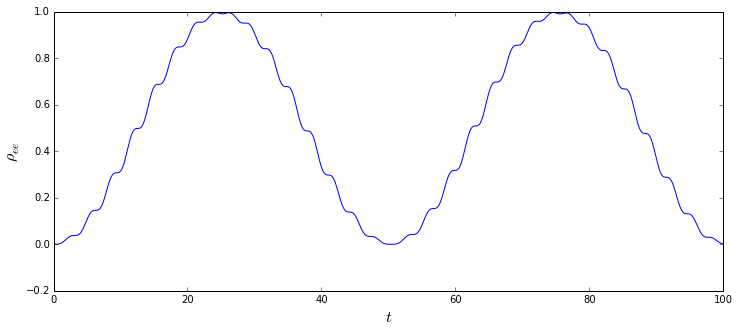We can see that only the two selected states are included in the dynamics, and very little leakage to other levels occur.

Instead of keeping all the inactive quantum states in the calculation we can eliminate them using Qobj.extract_state, so that we obtain a true two-level system.

In :
where(abs(ekets.full().flatten()) > 0.1)

Out:
array([10, 11])
In :
where(abs(ekets.full().flatten()) > 0.1)

Out:
array([10, 11])
In :
keep_states = where(abs(ekets.full().flatten()) > 0.1)

In :
H0 = H0.extract_states(keep_states)

H0

Out:
Quantum object: dims = [, ], shape = [2, 2], type = oper, isherm = True\begin{equation*}\left(\begin{array}{*{11}c}2.406\times10^{-04} & 0.496\\0.496 & 2.406\times10^{-04}\\\end{array}\right)\end{equation*}
In :
Hd = Hd.extract_states(keep_states)

Hd

Out:
Quantum object: dims = [, ], shape = [2, 2], type = oper, isherm = True\begin{equation*}\left(\begin{array}{*{11}c}0.125 & 0.0\\0.0 & -0.125\\\end{array}\right)\end{equation*}

And if we look at the energy level diagram now we see that we only have two states in the system, as desired.

In :
energy_level_diagram([H0, Hd], figsize=(4,2));In :
Heff = [H0, [Hd, 'sin(wd*t)']]

args = {'wd': (evals-evals)}

In :
psi0 = psi0.extract_states(keep_states)

In :
psi_e = psi_e.extract_states(keep_states)

In :
tlist = np.linspace(0.0, 100.0, 500)
result = mesolve(Heff, psi0, tlist, [], [ket2dm(psi_e)], args=args)

In :
visualize_dynamics(result, r'$\rho_{ee}$');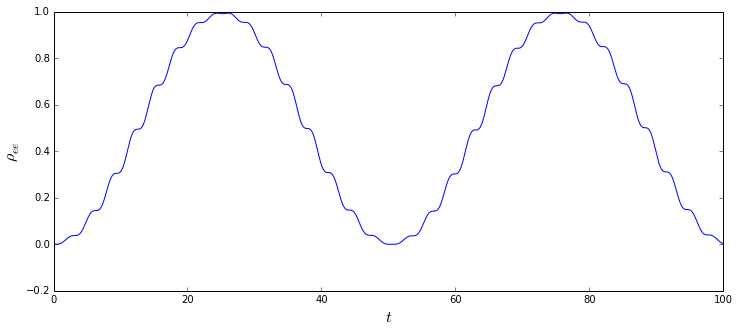### Software versions¶

In :
from qutip.ipynbtools import version_table; version_table()

Out:
SoftwareVersion
IPython2.0.0
Cython0.20.1post0
OSposix [linux]
Python3.4.1 (default, Jun 9 2014, 17:34:49) [GCC 4.8.3]
SciPy0.13.3
Numpy1.8.1
matplotlib1.3.1
QuTiP3.0.0.dev-5a88aa8
Thu Jun 26 14:05:41 2014 JST